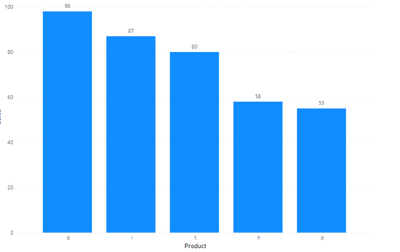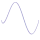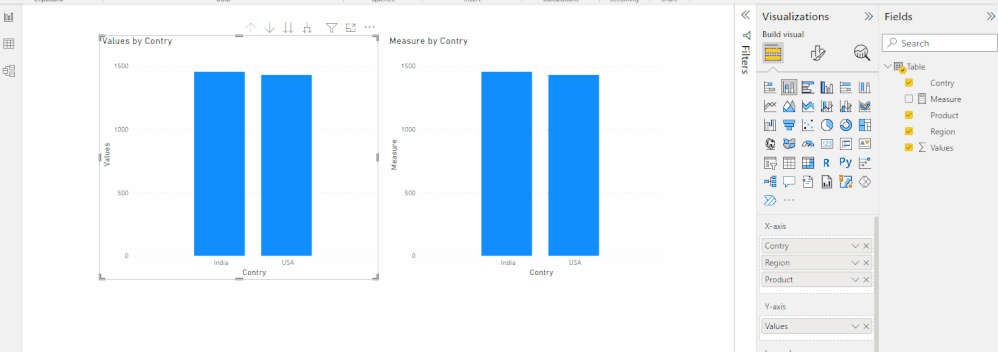cancel
Showing results for
Did you mean:Frequent Visitor

## Display Top 3 products on Drill Down

Hi Everyone!

Greetings of the day.

I require some help on the Scenario below:

I am displaying Sales by Country, Region and Product in a Bar Chart. But the User requirement is such that on drilling down at the Product Level, they only want the Top 3 Products, However the catch is that at an overall level (Country, Region) they would want to see the complete Sales for that particular dimension i.e Country & Region.

1.  At the Country level this is what they want, so no issues here.2. On drilling Down to a particular country say India at the Region level, this is fine as well.3. But on drilling to the Product level say for West Region, they only want the top 3 Products which is d,l,t is this case and not all products.Requesting you support on the Scenario above.

Thanks and Best Regards!!

1 ACCEPTED SOLUTIONCommunity Support

Hi @Ritvik777 ,

``````Measure =
VAR _c3 =
CALCULATETABLE (
TOPN (
3,
VALUES ( 'Table'[Contry] ),
CALCULATE ( SUM ( 'Table'[Values] ) ), DESC
),
ALLSELECTED ( 'Table' )
)
VAR _r3 =
CALCULATETABLE (
TOPN (
3,
VALUES ( 'Table'[Region] ),
CALCULATE ( SUM ( 'Table'[Values] ) ), DESC
),
ALLSELECTED ( 'Table' )
)
VAR _p3 =
CALCULATETABLE (
TOPN (
3,
VALUES ( 'Table'[Product] ),
CALCULATE ( SUM ( 'Table'[Values] ) ), DESC
),
ALLSELECTED ( 'Table' )
)
VAR _c =
IF ( SELECTEDVALUE ( 'Table'[Contry] ) IN _c3, SUM ( 'Table'[Values] ) )
VAR _r =
IF ( SELECTEDVALUE ( 'Table'[Region] ) IN _r3, SUM ( 'Table'[Values] ) )
VAR _p =
IF ( SELECTEDVALUE ( 'Table'[Product] ) IN _p3, SUM ( 'Table'[Values] ) )
RETURN
SWITCH (
FALSE (),
ISBLANK ( SELECTEDVALUE ( 'Table'[Contry] ) ), _c,
ISBLANK ( SELECTEDVALUE ( 'Table'[Region] ) ), _r,
ISBLANK ( SELECTEDVALUE ( 'Table'[Product] ) ), _p
)
``````

Result:Pbix in the end you can refer.

Best Regards

Community Support Team _ chenwu zhu

If this post helps, then please consider Accept it as the solution to help the other members find it more quickly.

3 REPLIES 3Community Support

Hi @Ritvik777 ,

``````Measure =
VAR _c3 =
CALCULATETABLE (
TOPN (
3,
VALUES ( 'Table'[Contry] ),
CALCULATE ( SUM ( 'Table'[Values] ) ), DESC
),
ALLSELECTED ( 'Table' )
)
VAR _r3 =
CALCULATETABLE (
TOPN (
3,
VALUES ( 'Table'[Region] ),
CALCULATE ( SUM ( 'Table'[Values] ) ), DESC
),
ALLSELECTED ( 'Table' )
)
VAR _p3 =
CALCULATETABLE (
TOPN (
3,
VALUES ( 'Table'[Product] ),
CALCULATE ( SUM ( 'Table'[Values] ) ), DESC
),
ALLSELECTED ( 'Table' )
)
VAR _c =
IF ( SELECTEDVALUE ( 'Table'[Contry] ) IN _c3, SUM ( 'Table'[Values] ) )
VAR _r =
IF ( SELECTEDVALUE ( 'Table'[Region] ) IN _r3, SUM ( 'Table'[Values] ) )
VAR _p =
IF ( SELECTEDVALUE ( 'Table'[Product] ) IN _p3, SUM ( 'Table'[Values] ) )
RETURN
SWITCH (
FALSE (),
ISBLANK ( SELECTEDVALUE ( 'Table'[Contry] ) ), _c,
ISBLANK ( SELECTEDVALUE ( 'Table'[Region] ) ), _r,
ISBLANK ( SELECTEDVALUE ( 'Table'[Product] ) ), _p
)
``````

Result:Pbix in the end you can refer.

Best Regards

Community Support Team _ chenwu zhu

If this post helps, then please consider Accept it as the solution to help the other members find it more quickly.Frequent Visitor

Hi Ritesh,

This helps.

But still looking for an exact solution for the business requirement.

Thanks for reaching out though.

Best Regards,

RitvikSuper UserAnnouncements#### Exclusive opportunity for Women!

Join us for a free, hands-on Microsoft workshop led by women trainers for women where you will learn how to build a Dashboard in a Day!#### Power Platform Conference-Power BI and Fabric Sessions

Join us Oct 1 - 6 in Las Vegas for the Microsoft Power Platform Conference.Top Solution Authors
Top Kudoed Authors
Users online (1,754)